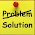## Saturday, 6 January 2018

### Python - Conditional Statement

One of the fairly common things in programming is making decision. For example comparing value and executing code based upon whether one value is greater than, less than or the same or not the same as another one. And that’s one condition comes into play.

In python programming language conditions are handle using if statement.

### Example:

```#!/usr/bin/python3

def main():
# working with conditional statements

x, y = 40,80
if (x < y):
compare_result = "x is less than y"
print(compare_result)
if __name__ == "__main__": main()`       `
```

### Code description

You can see here what is happening we are comparing x to y, and if x is less than y then the value of compare_result will be set as "x is less than y". And in the last we are printing this.

You will notice here that we are printing the compare_result variable outside if condition. When our condition goes True, the variable compare_result will be assigned the string ("x is less than y") and print statement will print out the value of variable compare_result.

Lest go ahead and run this, what would happen? You can see that x is less than y.

Let's see what would happened if we change the value of x from 40 to 100.

```#!/usr/bin/python3

def main():
# working with conditional statements

x, y = 100,80
if (x < y):
compare_result = "x is less than y"
print(compare_result)
if __name__ == "__main__": main()`   `
```

Here nothing happen we actually get an error because the line inside if condition never executed because x is not less than y, its greater than y, so print statement trying to print the value of variable that’s would never declared. That’s why we have an error.

Now we are going to figure out how to overcome this issue. To resolve this we need else statement. The purpose of using else statement is that, if the condition goes FALSE then execute else statement.

```#!/usr/bin/python3

def main():
# working with conditional statements

x, y = 100,80
if (x < y):
compare_result = "x is less than y"
else:
compare_result = "x is greater than y"
print(compare_result)
if __name__ == "__main__": main()```

And now we are getting here x is greater than y as an output.

Let's try some special case what would be happen if we try x is equal to y.

```#!/usr/bin/python3

def main():
# working with conditional statements

x, y = 80,80
if (x < y):
compare_result = "x is less than y"
else:
compare_result = "x is greater than y"
print(compare_result)
if __name__ == "__main__": main()```
```
```

The output is x is greater than y, but actually its not true they are the same value. The problem is that the if condition is evaluating to FALSE therefore else condition is evaluating even those it does not check that the x is greater than y or not. Lest fix that problem.

```#!/usr/bin/python3

def main():
# working with conditional statements

x, y = 80,80
if (x < y):
compare_result = "x is less than y"
elif (x == y):
compare_result = "x is same as y"
else:
compare_result = "x is greater than y"
print(compare_result)
if __name__ == "__main__": main()```
```
```

In above code snippet you have seen that now we have if, elif and else. So we have a way of chaining more than one condition together. If you are familiar to the JavaScript language you probably use to seeing it is written as if elseif and than elif. So JavaScript just takes if and else and combine them into one operator. Python has the elif operator right here (if, elif and else). So here we are checking the special case if x is equal to y rather than just x is greater than y. Let's Run above code snippet.

### Inline Conditional Statement

Python provide one another way of writing conditional statement. If you have only two situation for example (if and else) than you can use inline conditional statement.

```#!/usr/bin/python3

def main():
# working with conditional statements

x, y = 40,80
# Inline Conditional Statement

# Syntax
# do this if Condition True else do that
compare_result = "x is less than y" if (x < y) else "x is greater than y"
print(compare_result)
if __name__ == "__main__": main() ```
```
```

### Code Description

In above code snippet we have inline condition that shows that, print the string "x is less than y" if condition is TRUE and if condition is FALSE print the string "x is greater than y". So the one line condensed down the above code which we have written using traditional if else.

So python gives you nice and concise way of writing a conditional statement instead of writing an if, elif and else.

1.1.